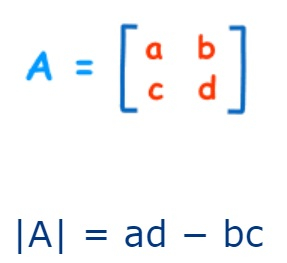# How can SciPy be used to calculate the determinant value of a matrix in Python?

The determinant value can be calculated on a matrix or on an array that has more than one dimension.

It may sometimes be required to understand the marix/array better. This is where the determinant operation would be needed.

SciPy offers a function named ‘det’ that is present in the ‘linalg’ class which is short for ‘Linear Algebra’.

Syntax of ‘det’ function

scipy.linalg.det(matrix)

The ‘matrix’ is the parameter that is passed to the ‘det’ function to find its determinant value.

This function can be called by passing the matrix/array as an argument.In the above picture, assume that ‘a’, ‘b’, ‘c’ and ‘d’ are numeric values of a matrix. The determinant is calculated by finding the difference between the product of ‘a’, ‘d’ and ‘b’,’c’.

Let us see how it can be done.

## Example

Live Demo

from scipy import linalg
import numpy as np
two_d_matrix = np.array([ [7, 9], [33, 8] ])
print("The determinant value of the matrix is :")
print(linalg.det(two_d_matrix ))

## Output

The determinant value of the matrix is :
-241.0

## Explanation

• The required libraries are imported.
• A matrix is defined with certain values in it.
• Parameters are passed to the ‘det’ function that computes the determinant value of the matrix.
• The function is called.
• This output is displayed on the console.